Courses

# The Integer Quantum Hall Effect (Part-3) Physics Notes | EduRev

## Physics : The Integer Quantum Hall Effect (Part-3) Physics Notes | EduRev

The document The Integer Quantum Hall Effect (Part-3) Physics Notes | EduRev is a part of the Physics Course Basic Physics for IIT JAM.
All you need of Physics at this link: Physics

An Aside: The Kubo Formula
Before we get to anything related to topology, we first need to lay some groundwork. Our task in this section will be to derive a formula for the Hall conductivity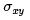in terms of quantum mechanical observables. The expression that we’re looking for is called the Kubo formula; it is part of more general story that goes by the name of linear response9.
We’ll derive the Kubo formula for a general, multi-particle Hamiltonian H0 where the subscript 0 means that this is the unperturbed Hamiltonian before we turn on an electric field. At this point, H0 could be that of many non-interacting particles each, for example, obeying the single-particle Hamiltonian (1.13) that we saw previously, or it could be something more complicated. Later, we’ll apply the Kubo formula both to Hamiltonians which describe particles moving in the continuum and to Hamiltonians that describe particles moving on a lattice. We denote the energy eigenstates of H0 as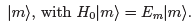Now we add a background electric field. We work in the gauge with At = 0 so that the electric field can be written as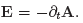. The new Hamiltonian takes the form H = H0 + ΔH with
ΔH = -J . A (2.8)
where J is the quantum operator associated to the electric current. For the simple Hamiltonians that we considered in Section 1.4, J is equal (up to constants) to the mechanical momentum π = p + eA = mx which we defined in equation (1.14). However, we’ll use more general definitions of J in what follows.
At this point, there’s a couple of tricks that makes life simpler. First, we’re ultimately interested in applying a constant, DC electric field. However, it turns out to be simpler to apply an AC electric field,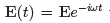with frequency ω, and to then take the limit ω→ 0. Second, it’s also somewhat simpler if we work with a complexified A. There’s nothing deep in this: it’s just easier to write e-iωt than, say, cos(ωt). Because all our calculations will be to linear order only, you can take the real part at any time. We then have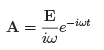(2.9)
Our goal is to compute the current (J) that flows due to the perturbation ΔH . We will assume that the electric field is small and proceed using standard perturbation theory.
We work in the interaction picture. This means that operators evolve as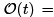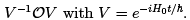In particular J, and hence ΔH (t) itself, both vary in time in this way. Meanwhile states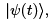evolve by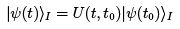where the unitary operator U (t; t0) is de ned as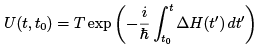(2.10)
Here T stands for time ordering; it ensures that U obeys the equation
We’re interested in systems with lots of particles. Later we’ll only consider noninteracting particles but, importantly, the Kubo formula is more general than this. We prepare the system at time t → — ∞ in a specific many-body state |0›. This is usually taken to be the many-body ground state, although it needn’t necessarily be. Then, writing U(t) = U(t,t→ -∞), the expectation value of the current is given by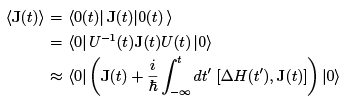where, in the final line, we’ve expanded the unitary operator (2.10), keeping only the leading terms. The first term is the current in the absence of an electric field. We’ll assume that this term vanishes. Using the expressions (2.8) and (2.9), the current due to the electric field is then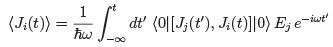Because the system is invariant under time translations, the correlation function above can only depend on t" = t — t'. We can then rewrite the expression above as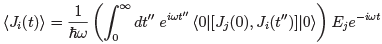The only t dependence in the formula above sits outside as e-iωt. This is telling us that if you apply an electric field at frequency u, the current responds by oscillating at the same frequency ω. This is the essence of linear response. The proportionality constant defines the frequency-dependent conductivity matrix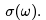. The Hall conductivity is the off-diagonal part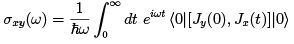This is the Kubo formula for the Hall conductivity.
We can massage the Kubo formula into a slightly more useful form. We use the fact that the current operator evolves as J(t) = V-1 J(0) V with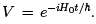. We then evaluate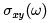by inserting complete basis of energy eigenstates of H0,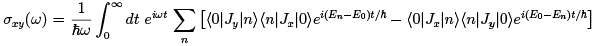We now perform the integral over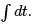(There’s a subtlety here: to ensure convergence, we should replace ω → ω + iϵ, with ϵ infinitesimal. There is a story related to causality and where poles can appear in the complex ω plane which you can learn more about in the Kinetic Theory lecture notes.) Since the states with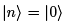don’t contribute to the sum, we get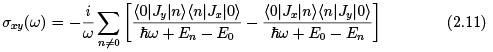Now, finally, we can look at the DC ω → 0 limit that we’re interested in. We expand the denominators as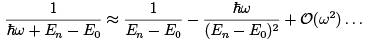and similar for the other term. The first term looks divergent. Indeed, such divergences do arise for longitudinal conductivities and tell us something physical, often that momentum is conserved due to translational invariance so there can be no DC resistivity. However, in the present case of the Hall conductivity, there is no divergence because this term vanishes. This can be shown on general grounds from gauge invariance or, equivalently, from the conservation of the current. Alternatively - although somewhat weaker - it can quickly seen by rotational invariance which ensures that the expression should be invariant under x → y and y → -x. We’re then left only with a finite contribution in the limit ω → 0 given by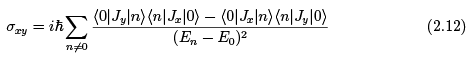This is the Kubo formula for Hall conductivity
Before we proceed, I should quickly apologise for being sloppy: the operator that we called J in (2.8) is actually the current rather than the current density. This means that the right-hand-side of (2.12) should, strictly speaking, be multiplied by the spatial area of the sample. It was simpler to omit this in the above derivation to avoid clutter.

The Role of Topology
In this section, we describe a set-up in which we can see the deep connections between topology and the Hall conductivity. The set-up is closely related to the gauge-invariance argument that we saw in Section 2.2.2. However, we will consider the Hall system on a spatial torus T2. This can be viewed as a rectangle with opposite edges identified. We’ll take the lengths of the sides to be Lx and Ly.
We thread a uniform magnetic field B through the torus. The first result we need is that B obeys the Dirac quantisation condition,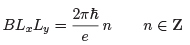(2.13)
This quantisation arises for the same reason that we saw in Section 1.5.2 when discussing the Berry phase. However, it’s an important result so here we give an alternative derivation.
We consider wavefunctions over the torus and ask: what periodicity requirements should we put on the wavefunction? The first guess is that we should insist that wavefunctions obey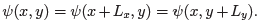. But this turns out to be too restrictive when there is a magnetic flux through the torus. Instead, one has to work in patches; on the overlap between two different patches, wavefunctions must be related by a gauge transformation.
Operationally, there is a slightly simpler way to implement this. We introduce the magnetic translation operators,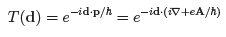These operators translate a state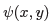by position vector d. The appropriate boundary conditions will be that when a state is translated around a cycle of the torus, it comes back to itself. So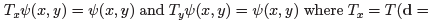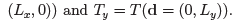It is clear from the expression above that the translation operators are not gauge invariant: they depend on our choice of A. We’ll choose Landau gauge Ax = 0 and Ay = Bx. With this choice, translations in the x direction are the same as those in the absence of a magnetic field, while translations in the y direction pick up an extra phase. If we take a state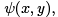translated around a cycle of the torus, it becomes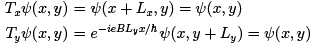Notice that we can see explicitly in the last of these equations that the wavefunction is not periodic in the naive sense in the y direction: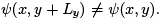. Instead, the two wavefunctions agree only up to a gauge transformation.
However, these equations are not consistent for any choice of B. This follows by comparing what happens if we translate around the x-cycle, followed by the y-cycle, or if we do these in the opposite order. We have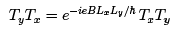(2.14)
Since both are required to give us back the same state, we must have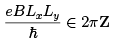This is the Dirac quantisation condition (2.13).
There is an interesting story about solving for the wavefunctions of a free particle on a torus in the presence of a magnetic field. They are given by theta functions. We won’t discuss these here.

Now we’re going to perturb the system. We do this by threading two fluxes, Φx and Φthrough the x and y-cycles of the torus respectively. This means that the gauge potential becomes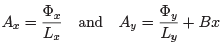This is the same kind of set-up that we discussed in Section 2.2.2; the only difference is that now the geometry allows us to introduce two fluxes instead of one. Just as in our previous discussion, the states of the quantum system are only sensitive to the non-integer part of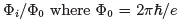is the quantum of flux. In particular, if we increase either Φi from zero to Φ0, then the spectrum of the quantum system must be invariant. However, just as in Section 2.2.2, the system can undergo spectral flow.
The addition of the fluxes adds an extra term to the Hamiltonian of the form (2.8),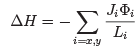We want to see how this affects the ground state of the system which we will denote as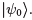. (We called this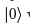when deriving the Kubo formula, but we’ll want to differentiate it soon and the expression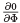just looks too odd!). We’ll assume that the ground state is nondegenerate and that there is a gap to the first excited state. Then, to first order in perturbation theory, the ground state becomes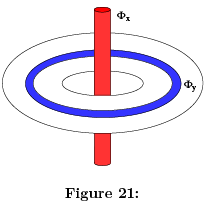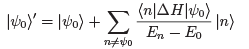Considering in finitesimal changes of Φi, we can write this as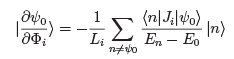But the right-hand-side is exactly the kind of expression that appeared in the Kubo formula (2.12). This means that, including the correct factors of the spatial area, we can write the Hall conductivity as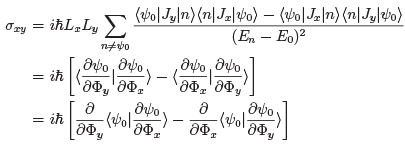As we now explain, this final way of writing the Hall conductivity provides a novel perspective on the integer quantum Hall effect.

Hall Conductivity and the Chern Number
The fluxes Φi appear as parameters in the perturbed Hamiltonian. As we discussed above, the spectrum of the Hamiltonian only depends on Φmod Φ0, which means that these parameters should be thought of as periodic: the space of the flux parameters is itself a torus,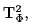where the subscript is there to distinguish it from the spatial torus that we started with. We'll introduce dimensionless angular variables,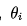to parameterise this torus,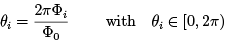As we discussed in Section 1.5, given a parameter space it is natural to consider the Berry phase that arises as the parameters are varied. This is described by the Berry connection which, in this case, lives over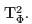It is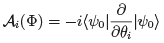The field strength, or curvature, associated to the Berry connection is given by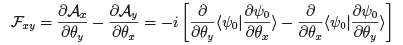This is precisely our expression for the Hall conductivity! We learn that, for the torus with fluxes, we can write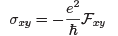This is a nice formula. But, so far, it doesn’t explain why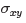is quantised. However, suppose that we average over all fluxes. In this case we integrate over the torus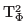of parameters to get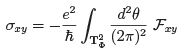The integral of the curvature over, is a number known as the first Chern number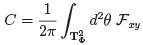Importantly, this is always an integer: C ∈ Z. This follows from the same kind of argument that we made in Section 1.5 (or, alternatively, the kind of argument that we made at the beginning of this section on Dirac quantisation). The net result is that if we average over the fluxes, the Hall conductivity is necessarily quantised as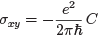(2.15)
This, of course, is the integer quantum Hall effect. The relationship between the Hall conductivity and the Chern number is usually referred to as the TKNN invariant (after Thouless, Kohomoto, Nightingale and den Nijs) although, strictly speaking, this name should be reserved for a very similar expression that we'll discuss in the next section.

Offer running on EduRev: Apply code STAYHOME200 to get INR 200 off on our premium plan EduRev Infinity!

## Basic Physics for IIT JAM

109 videos|157 docs|94 tests

,

,

,

,

,

,

,

,

,

,

,

,

,

,

,

,

,

,

,

,

,

;